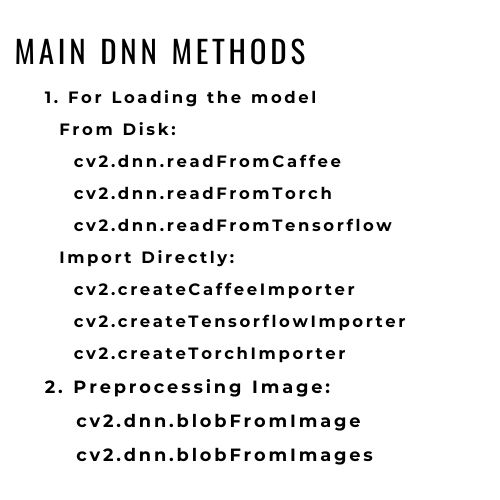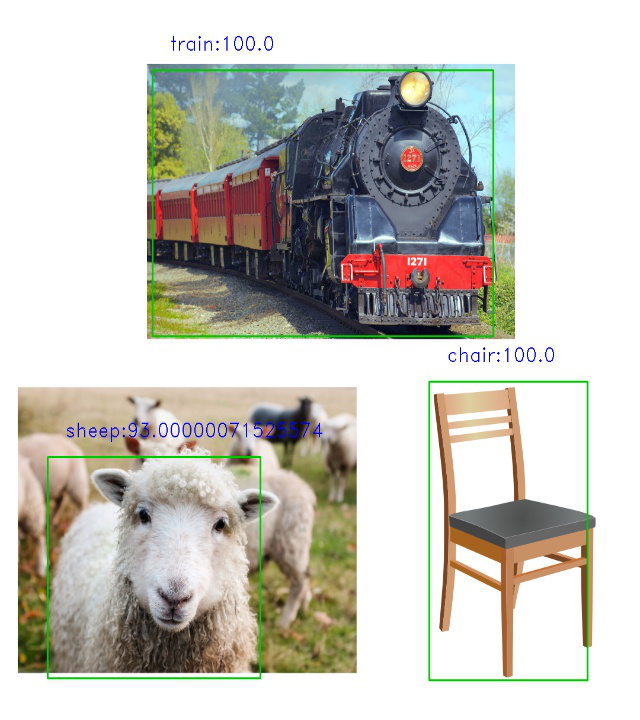GFG App
Open AppBrowser
Continue

# Deep Learning with Python OpenCV

Opencv 3.3 brought with a very improved and efficient (dnn) module which makes it very for you to use deep learning with OpenCV. You still cannot train models in OpenCV, and they probably don’t have any intention of doing anything like that, but now you can very easily use image processing and use the pre-trained models to make predictions using the dnn module.

This new version supports a number of large frameworks which include :

1. Tensorflow
2. Torch
3. Caffe

## Objective

In this article, we’ll walk you through the entire process of using a pre-trained model, loading it using the dnn module, image preprocessing using the blobfromImage method in OpenCV, and then finally making predictions.

There are two ways to load models from frameworks in OpenCV :

1. If you want to import the model directly, then use the cv2.dnn.createCaffeImporter or change the caffe to Torch or Tensorflow, depending on which framework you’re using.
2. If you want to load from the disk then use the cv2.dnn.readNetFromCaffeWe’ll be taking the Mobile_net_ssd caffe model for object detection as an example to understand how dnn module works. And we’ll be using the second approach i.e downloading the model files and loading the model using the dnn module.

`pip install opencv-python dlib imutils`

Since we’re using caffe model we’ll use the cv2.dnn.readNetFromCaffe module to load our model. You will need these two types of files to work with any pre-trained model using dnn module:

1. .prototxt file: They basically contain a list of the network layers in the model that you’re using.
2. caffemodel file (in your case it might not be a Caffe model): This file contains the weights of the model.

You need both of these files to create the model, we’ll pass these two files as arguments to the cv2.dnn.readNetFromCaffe module to create our model.

#—–Paths of the model files——–#

proto_file = ‘Model/MobileNetSSD_deploy.prototxt.txt’

model_file = ‘Model/MobileNetSSD_deploy.caffemodel’

Now that we’ve our file paths, we’ll load our model:

Before using this model for prediction we’ve to preprocess our image to set it to the requirements of our model input, and this differs from model to model.

## Image Preprocessing

So we’ll define a few variables for image preprocessing.

#——Class Labels of the model——–#

classNames = { 0: ‘background’,

1: ‘aeroplane’, 2: ‘bicycle’, 3: ‘bird’, 4: ‘boat’,

5: ‘bottle’, 6: ‘bus’, 7: ‘car’, 8: ‘cat’, 9: ‘chair’,

10: ‘cow’, 11: ‘diningtable’, 12: ‘dog’, 13: ‘horse’,

14: ‘motorbike’, 15: ‘person’, 16: ‘pottedplant’,

17: ‘sheep’, 18: ‘sofa’, 19: ‘train’, 20: ‘tvmonitor’ }

#——–Scaling parameters——#

input_shape=(300,300) #the required shape for the input image to pass to our model

mean = (127.5,127.5,127.5) #we’ll have to normalize the image pixels, and we’ll use this mean value to do that

scale = 0.007843 # then finally we’ll scale the image to meet the input criteria of the model

The dnn module provides us with the blobFromImage( or blobFromImages if you’re using multiple images) method for the pre-processing steps and we just have to pass the scaling parameters we defined above to complete the preprocessing step, and get the required blob i.e input image.

#——image preprocessing—-#

blob = cv2.dnn.blobFromImage(img,

scalefactor=scale,

size=input_shape,

mean=mean,

swapRB=True) #since our image is already in the BGR form because opencv by default reads it in BGR format

## Make Predictions using the model

Now that we have our input ready, we have to explicitly set it as input using the setInput() method and then pass it to our model and use the forward method to generate predictions.

#——setting input—–#

net.setInput(blob)

#—–using the model to make predictions

results = net.forward()

The forward method returns us a 4-dimensional list:

The 3rd dimension has our predictions, and each prediction is a list of 7 floating values. At the 1 index we have the class_id, at 2nd index we have the confidence/probability and from 3rd to 6th index we have the coordinates of the object detected.

Let’s directly see how they are used in our final implementation.

## Python3

 `import` `cv2` `import` `dlib` `from` `imutils ``import` `face_utils`   `img ``=` `cv2.imread(``'object (1).png'``)`   `#--------Model Path---------#` `proto_file ``=` `'SSD_MobileNet_prototxt.txt'` `model_file ``=` `'SSD_MobileNet.caffemodel'`   `#------Variables for the Model ---------#` `classNames ``=` `{``0``: ``'background'``,` `              ``1``: ``'aeroplane'``, ``2``: ``'bicycle'``, ` `              ``3``: ``'bird'``, ``4``: ``'boat'``,` `              ``5``: ``'bottle'``, ``6``: ``'bus'``, ``7``: ``'car'``,` `              ``8``: ``'cat'``, ``9``: ``'chair'``,` `              ``10``: ``'cow'``, ``11``: ``'diningtable'``, ` `              ``12``: ``'dog'``, ``13``: ``'horse'``,` `              ``14``: ``'motorbike'``, ``15``: ``'person'``, ` `              ``16``: ``'pottedplant'``,` `              ``17``: ``'sheep'``, ``18``: ``'sofa'``, ` `              ``19``: ``'train'``, ``20``: ``'tvmonitor'``}`   `input_shape ``=` `(``300``, ``300``)` `mean ``=` `(``127.5``, ``127.5``, ``127.5``)` `scale ``=` `0.007843`   `#---------Load The Model--------#` `net ``=` `cv2.dnn.readNetFromCaffe(proto_file, model_file)`   `#------image preprocessing----#` `blob ``=` `cv2.dnn.blobFromImage(img,` `                             ``scalefactor``=``scale,` `                             ``size``=``input_shape,` `                             ``mean``=``mean,` `                             ``swapRB``=``True``)  ` `# since our image is already in the BGR form`   `net.setInput(blob)` `results ``=` `net.forward()` `for` `i ``in` `range``(results.shape[``2``]):` `  `  `      ``# confidence` `    ``confidence ``=` `round``(results[``0``, ``0``, i, ``2``],``2``) ` `    ``if` `confidence > ``0.7``:` `      `  `          ``# class id` `        ``id` `=` `int``(results[``0``, ``0``, i, ``1``])  ` `        `  `        ``# 3-6 contains the coordinate` `        ``x1, y1, x2, y2 ``=` `results[``0``, ``0``, i, ``3``:``7``]  ` `        `  `        ``# print(x1,y1,x2,y2)` `        ``# scale these coordinates to out image pixel` `        ``ih, iw, ic ``=` `img.shape` `        ``x1, x2 ``=` `int``(x1``*``iw), ``int``(x2``*``iw)` `        ``y1, y2 ``=` `int``(y1 ``*` `ih), ``int``(y2 ``*` `ih)` `        ``cv2.rectangle(img,` `                      ``(x1, y1),` `                      ``(x2, y2),` `                      ``(``0``, ``200``, ``0``), ``2``)` `        ``cv2.putText(img, f``'{classNames[id]}:{confidence*100}'``,` `                    ``(x1``+``30``, y1``-``30``), ` `                    ``cv2.FONT_HERSHEY_DUPLEX, ` `                    ``1``, (``255``, ``0``, ``0``), ``1``)` `    ``# print(results[0,0,i,:])`   `img ``=` `cv2.resize(img, (``640``, ``720``))` `cv2.imshow(``'Image'``, img)` `# cv2.imwrite('output1.jpg',img) # Uncomment this line to save the output` `cv2.waitKey()`

Output:## What next?

Now that you know how to use pre-trained models, try to use various pre-trained models from different frameworks and create different applications like language translation, image segmentation, style transfer etc.

My Personal Notes arrow_drop_up